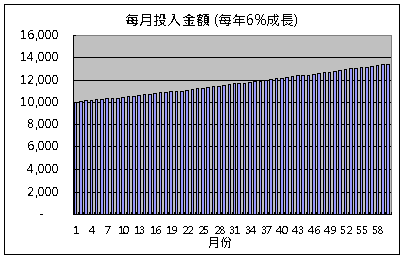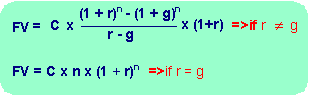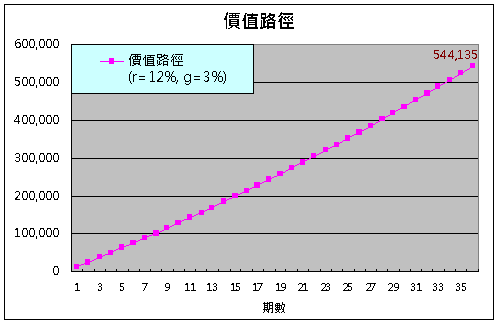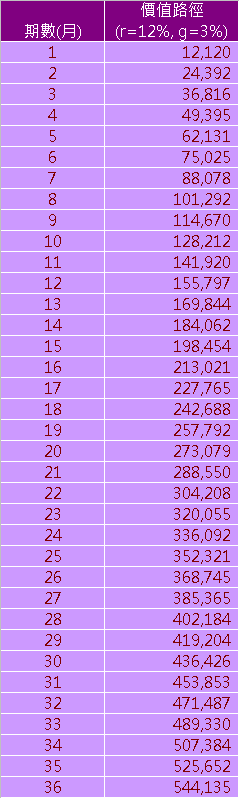定期定值之理論基礎

﻿

1. 預期投資金額會隨著時間而成長
2. 每期金額會依目前績效偏離目標之程度而動態調整

貨幣時間價值

▼第一次投入1萬元，每年以6%成長期末終值計算公式

▼公式(一)▼公式(二)=(5000000*(1%-0.5%))/((1+1%)*((1+1%)^120-(1+0.5%)^120))
=16,714

= 5000000/(120*(1+0.75%)^120)
= 16,997

價值路徑

▼投入金額年成長率3%、年報酬率12%，第一期投入12,000之價值路徑圖多退少補策略

=24392-213.9075*55.94 = 12,426

=12426/55.94
=222.1309

參考資料

﻿

■ 本網站內容儘可能精確完整，但不保證無誤。若做為投資依據，風險請自行斟酌 ，本網站不負賠償之責任。
■ 網站所有資料均為版權所有，非經書面允許請勿轉載或使用。